본문바로가기

## 검색결과

검색결과 (전체 1건)

### 검색결과제한

열기
• 소장도서관
• 원문
• 발행년도
-
• 언어
• 원문이용안내
•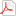모든 이용자
•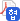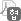협정기관 이용자
•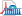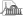국회도서관 방문 이용자
• 원문아이콘이 없는 자료는 국회도서관에 방문하여 책자로만 이용이 가능합니다.

논문명/저자명
Rhodococcus spp.에 의한 hexane 및 cyclohexane의 생분해에 관한 연구 / 이은희
발행사항
서울 : 이화여자대학교 과학기술대학원, 2006.2
청구기호
TM 628.53 ㅇ824r
형태사항
xx, 241 p. ; 26 cm
자료실
전자자료
제어번호
KDMT1200632692
주기사항
학위논문(석사) -- 이화여자대학교 과학기술대학원, 환경학, 2006.2
원문
미리보기

목차보기더보기

국문목차

표제지=0,1,4

목차=i,5,7

표목차=viii,12,4

그림목차=xii,16,7

감사의 글=xix,23,2

제1장 Hexane 분해 균주 분리 및 특성화에 관한 연구=1,25,1

논문개요=1,25,2

I. 서론=3,27,2

II. 이론적 배경=5,29,1

2.1. Hexane의 특징=5,29,1

2.1.1. Hexane의 물리 화학적 특징=5,29,4

2.2. Hexane의 위해성=9,33,1

2.2.1. 광화학 스모그 유발=9,33,2

2.2.2. Hexane의 생물체에 대한 위해성=10,34,1

2.2.2.1. Hexane의 미생물에 대한 독성=10,34,2

2.2.2.2. Hexane의 생물에 대한 독성=11,35,2

2.2.2.3. Hexane의 인체에 대한 영향=12,36,3

2.3. Hexane의 오염원=15,39,1

2.3.1. Hexane 사용현황=15,39,2

2.3.2. Hexane 배출원=16,40,4

2.3.3. 환경에서의 hexane 자연거동(environmental fate)=19,43,2

2.4. Hexane 규제 현황=21,45,1

2.4.1. Hexane 규제=21,45,2

2.5. Hexane 분해 기작=23,47,2

2.6. 연구목적=25,49,1

III. 실험재료 및 방법=26,50,1

3.1. Hexane 분해 미생물 consortia 개발=26,50,1

3.1.1. Hexane 분해 세균 농화배양(enrichment culture)=26,50,1

3.1.2. Hexane 분해용 consortia의 미생물 군집 분석=26,50,4

3.1.3. Hexane 분해 consortia 활성 분석=29,53,2

3.1.4. 14C-hexane을 이용한 hexane 생분해 실험(이미지참조)=30,54,2

3.2. 분석 방법=31,55,1

3.2.1. 기질 분석 방법=31,55,2

3.3. Hexane 분해 균주 순수 분리=32,56,1

3.3.1. Hexane 분해 균주의 분리 및 배양=32,56,2

3.3.2. Hexane 분해 순수균의 동정=33,57,2

3.4. Hexane 분해 순수균의 활성 조사=34,58,1

3.4.1. Hexane 분해 순수균의 활성 실험=34,58,1

3.4.2. 균주의 건조중량과 탁도의 상관관계 도출=34,58,2

3.4.3. 14C-Hexane 이용한 순수균 생분해 실험(이미지참조)=35,59,1

3.5. Hexane 분해 경로 조사=35,59,1

3.5.1. Hexane 중간 대사산물 분석 및 동정=35,59,2

3.6. 물리적 환경 인자에 따른 hexane 분해 활성 실험=37,61,1

3.6.1. 온도영향=37,61,1

3.6.2. pH 영향=37,61,1

3.6.3. 염농도 영향=38,62,1

3.7. 타 탄화수소화합물 이용가능성=38,62,2

3.7.1. 난분해성 탄화수소화합물 분해가능성=39,63,1

3.7.2. BTEX와 MTBE 생분해에 미치는 기질 상호관계=40,64,1

3.8. 통계 분석=40,64,1

IV. 결과 및 고찰=41,65,1

4.1. Hexane 분해 미생물 consortia 개발=41,65,1

4.1.1. Hexane 분해 세균 농화 배양=41,65,1

4.1.2. Consortia의 미생물 군집 구조 분석=42,66,6

4.1.3. Consortia의 미생물 성장 속도 측정=48,72,3

4.1.4. Consortia의 hexane 분해 활성=51,75,3

4.1.5. 14C-hexane을 이용한 consortia의 hexane 분해산물 분석(이미지참조)=53,77,2

4.2. 순수균의 분리 및 동정=54,78,1

4.2.1. Hexane 분해 순수균 분리=54,78,2

4.2.2. 균의 동정=55,79,4

4.3. Hexane 분해 순수균의 활성 실험=59,83,1

4.3.1. Hexane 분해 순수균의 성장 속도 측정=59,83,2

4.3.2. Hexane 분해 순수균의 hexane 분해능 조사=61,85,3

4.3.3. Hexane 분해 순수균의 hexane 무기화=63,87,2

4.4. Hexane 분해 경로 조사=64,88,1

4.4.1. Hexane 분해 중간대사산물 분석=64,88,2

4.5. 물리적 환경 인자에 따른 hexane 분해 활성 실험=65,89,1

4.5.1. 온도 영향=65,89,3

4.5.2. pH 영향=67,91,2

4.5.3. 염 농도 영향=68,92,2

4.6. EH831의 타 탄화수소화합물 이용 가능성=69,93,1

4.6.1. 탄화수소화합물 이용 가능성=69,93,3

4.6.2. 타 난분해성 탄화수소화합물의 분해 특성=71,95,1

4.6.2.1. EH831의 MTBE 생분해 특성=72,96,5

4.6.2.2. EH831의 dichloromethane 생분해 특성=76,100,3

4.6.2.3. EH831의 BTEX 생분해 특성=78,102,9

4.6.2.4. EH831의 cyclohexane 생분해 특성=86,110,6

4.6.3. EH831의 BTEX와 MTBE 생분해에 미치는 기질 상호관계=91,115,47

V. 결론=138,162,3

참고문헌=141,165,29

Abstract=170,194,2

제2장 Cyclohexane 분해균주 분리 및 특성화에 관한 연구=172,196,1

논문개요=172,196,2

I. 서론=174,198,2

II. 이론적 배경=176,200,1

2.1. Cyclohexane의 특징=176,200,1

2.1.1. Cyclohexane의 물리 화학적 특징=176,200,2

2.2. Cyclohexane의 독성=177,201,1

2.2.1. 세포막에 대한 cyclohexane의 독성=177,201,4

2.2.2. 미생물에 대한 cyclohexane의 독성=180,204,3

2.2.3. 고등생물에 대한 cyclohexane의 독성=183,207,2

2.3. 미생물에 의한 cyclohexane 생분해 특성=185,209,1

2.3.1. Cyclohexane 생분해 미생물=185,209,2

2.3.2. Cyclohexane 생분해 기작=186,210,2

2.4. 연구 목적=187,211,2

III. 실험재료 및 방법=189,213,1

3.1. Cyclohexane 분해 미생물 consortium 개발=189,213,1

3.1.1. Cyclohexane 분해 세균 농화배양(enrichment culture)=189,213,1

3.1.2. Cyclohexane 분해용 consortium의 미생물 군집 분석=189,213,1

3.1.3. Cyclohexane 분해 consortium 활성 분석=189,213,1

3.2. 기질 분석 방법=189,213,1

3.3. Cyclohexane 분해 균주 순수 분리=189,213,1

3.3.1. Cyclohexane 분해 균주의 분리 및 배양=189,213,2

3.3.2. Cyclohexane 분해 순수균의 동정=190,214,1

3.4. Cyclohexane 분해 순수균의 활성 조사=190,214,1

3.4.1. Cyclohexane 분해 순수균의 활성 실험=190,214,1

3.4.2. 균주의 건조중량과 탁도의 상관관계 도출=190,214,1

3.4.3. 14C-hexane을 이용한 순수균 생분해 실험(이미지참조)=190,214,1

3.5. Cyclohexane 생분해 경로 조사=191,215,1

3.5.1. Cyclohexane 중간 대사산물 분석 및 동정=191,215,1

3.6. 물리적 환경 인자에 따른 cyclohexane 분해 활성 실험=191,215,1

3.6.1. 온도영향=191,215,1

3.6.2. pH 영향=191,215,1

3.6.3. 염농도 영향=191,215,1

3.7. 타 탄화수소 화합물 이용가능성=191,215,1

3.8. 통계 분석=192,216,1

IV. 결과 및 고찰=193,217,1

4.1. Cyclohexane 분해 미생물 consortium 개발=193,217,1

4.1.1. Cyclohexane 분해 세균 농화 배양=193,217,1

4.1.2. Consortium OCC의 미생물 군집 구조 분석=194,218,3

4.1.3. Consortium OCC의 미생물 성장속도 측정=196,220,2

4.2. Cyclohexane 분해 순수균의 분리 및 동정=198,222,1

4.2.1. Cyclohexane 분해 순수균 분리=198,222,1

4.2.2. 순수균의 동정=198,222,2

4.3. Cyclohexane 분해 순수균의 활성 실험=199,223,1

4.3.1. Cyclohexane 분해 순수균의 성장 속도 측정=199,223,4

4.3.2. Cyclohexane 분해 순수균의 분해능 조사=202,226,4

4.3.3. EC1의 hexane 무기화=205,229,2

4.4. Cyclohexane 생분해 경로 조사=206,230,1

4.4.1. Cyclohexane 분해 중간 대사산물 분석=206,230,2

4.5. 물리적 환경 인자에 따른 cyclohexane 분해 활성 실험=207,231,1

4.5.1. 온도 영향=207,231,2

4.5.2. pH 영향=208,232,2

4.5.3. 염농도 영향=209,233,2

4.6. EC1의 타 탄화수소화합물 이용 가능성=210,234,1

4.6.1. 탄화수소화합물 이용 가능성=210,234,3

V./(VI) 결론=213,237,3

참고문헌=216,240,6

부록=222,246,18

Abstract=240,264,2

Table1.2.1. The regulated volatile organic compounds in Korea=7,31,1

Table1.2.2. The physico-chemical properties of n-hexane=8,32,1

Table1.2.3. Comparison of Photochemical Ozone Potential(POCP)values=10,34,1

Table1.2.4. Hexane ecotoxicity information=12,36,1

Table1.2.5. Hexane potential health effects=14,38,1

Table1.2.6. The emission and transport amounts of n-hexane=17,41,1

Table1.2.7. The emission and transport amounts of n-hexane according to the industries=17,41,1

Table1.2.8. Comparison of local emission and transport amounts of n-hexane=18,42,1

Table1.2.9. Comparison of emission and transport amounts of n-hexane by industry complex=19,43,1

Table1.2.10. The reaction rate and half-life of hexane and chlorinated VOCs=20,44,1

Table1.2.11. Bioconcentration factors for media to community receptors=20,44,1

Table1.2.12. Guidelines applicable to n-hexane=22,46,1

Table1.3.1. 1st PCR condition=27,51,1

Table1.3.2. 2nd PCR condition=28,52,1

Table1.3.3. The compositions of pH buffer solution=37,61,1

Table1.3.4. The compositions of LB medium with different NaCl concentration=38,62,1

Table1.3.5. The injection amounts of substrates=39,63,1

Table1.3.6. Experimental conditions for the interaction test of BTEX and MTBE=40,64,1

Table1.4.1. Summary of phylogenetic diversity of hexane-degrading consortium OH based on 16S rDNA sequences=45,69,1

Table1.4.2. Summary of phylogenetic diversity of hexane-degrading consortium CH based on 16S rDNA sequences=47,71,1

Table1.4.3. Comparisons of hexane degradation rate and specific growth rate=50,74,1

Table1.4.4. Mass balance results for 14C portion in the serum bottle after five days' growth(이미지참조)=54,78,1

Table1.4.5. The sequences and identification of strains EH741 and EH831=58,82,1

Table1.4.6. Comparisons of hexane degradation rate and growth rate=59,83,1

Table1.4.7. Mass balance results for 14C portion in the serum bottle after five days' growth(이미지참조)=64,88,1

Table1.4.8. Utilization of alternative substrates by EH831=71,95,1

Table1.4.9. Comparison of kinetic parameters obtained with selected literature values=85,109,2

Table1.4.10. Comparison of degradation rate of various compounds by EH831=91,115,1

Table1.4.11. Effects of BTEXM mixtures on the benzene degradation rate by EH831=111,135,1

Table1.4.12. Effects of BTEXM mixtures on the toluene degradation rate by EH831=112,136,1

Table1.4.13. Effects of BTEXM mixtures on the ethylbenzene degradation rate by EH831=113,137,1

Table1.4.14. Effects of BTEXM mixtures on the xylenes degradation rate by EH831=114,138,1

Table1.4.15. Effects of BTEXM mixtures on the MTBE degradation rate of EH831=115,139,1

Table1.4.16. Comparison of removal amount of each gas in the mixtures of B, T, E, X, and M=121,145,2

Table2.2.1. Physico-chemical properties of cyclohexane=177,201,1

Table2.2.2. Organic solvents and their log P values=181,205,1

Table2.2.3. Effects of increasing concentrations of cyclohexane on mitochondrial membrane potential by using ethanol or succinate as a substrate=182,206,1

Table2.2.4. Effects of different concentrations of cyclohexane on mitochondrial ATP synthesis and ATPase activity=182,206,1

Table2.2.5. Cyclohexane potential health effects(U.S. EPA, 1994)=183,207,1

Table2.2.6. Cyclohexane toxicological information(U.S. EPA, 1994)=184,208,1

Table2.2.7. Cyclohexane ecological information(U.S. EPA, 1994)=184,208,1

Table2.4.1. Summary of phylogenetic diversity of cyclohexane-degrading consortium OCC based on 165 rDNA sequences=196,220,1

Table2.4.2. Cyclohexane specific growth rate of consortium OCC=197,221,1

Table2.4.3. The sequences and identification of strain EC1=199,223,1

Table2.4.4. Cyclohexane or hexane degradation rates and specific growth rates by EC1=202,226,1

Table2.4.5. Mass balance results for 14C portion in the serum bottle after five days' growth(이미지참조)=206,230,1

Table2.4.6. Utilization of alternative substrates by EC1=212,236,1

Fig.1.2.1. Common uses of n-hexane in Korea, 2002(화학경제연구원, 2003)=16,40,1

Fig.1.2.2. Proposed pathway for the degradation of n-hexane by the denitrifying bacterium strain HxN1=24,48,1

Fig.1.4.1. The biodegradation of hexane by consortia=41,65,1

Fig.1.4.2. Characterization of bacterial community in consortium OH=44,68,1

Fig.1.4.3. Characterization of bacterial community in consortium CH=46,70,1

Fig.1.4.4. Growth of the consortium OH in the liquid media containing different concentrations of hexane=48,72,1

Fig.1.4.5. Growth of the consortium CH in the liquid media containing different concentrations of hexane=49,73,1

Fig.1.4.6. The Lineweaver-Burk plot of specific growth rate by consortium OH and consortium CH=50,74,1

Fig.1.4.7. Hexane degradation by consortium OH in the liquid media containing different concentrations of hexane=51,75,1

Fig.1.4.8. Hexane degradation by consortium CH in the liquid media containing different concentrations of hexane=52,76,1

Fig.1.4.9. The Lineweaver-Burk plot of specific degradation rate by consortium OH and consortium CH=53,77,1

Fig.1.4.10. Comparison of hexane degradation by isolates=55,79,1

Fig.1.4.11. Effect of surfactant addition on the cell growth of EH741=57,81,1

Fig.1.4.12. Growth of the isolates in the liquid medium containing different concentrations of hexane=60,84,1

Fig.1.4.13. (a)Relationship between hexane concentration and specific growth rate.(b)The Linweaver-Burk plot=60,84,1

Fig.1.4.14. Hexane degradation by isolates in the liquid medium containing different concentrations of hexane=62,86,1

Fig.1.4.15. (a)Relationship between hexane concentration and specific degradation rate.(b)The Lineweaver-Burk plot=63,87,1

Fig.1.4.16. Gas-chromatograph of gas sampled from headspace in the closed bottle inoculated with EH831=65,89,1

Fig.1.4.17. Hexane degradability of EH831 at different temperatures=66,90,1

Fig.1.4.18. Effects of temperature on hexane degradation rate of EH831=67,91,1

Fig.1.4.19. Effects of pH on hexane degradation rate of EH83l=68,92,1

Fig.1.4.20. Effects of salt concentration on the growth rate of EH831=69,93,1

Fig.1.4.22. The biodegradation of DCM by strain EH831=78,102,1

Fig.1.4.23. The biodegradation of benzene by EH831=79,103,1

Fig.1.4.24. The biodegradation of toluene by EH831=80,104,1

Fig.1.4.25. The biodegradation of ethylbenzene by EH831=81,105,1

Fig.1.4.26. The biodegradation of all isomer xylenes by EH831=82,106,1

Fig.1.4.27. Cyclohexane degradation by EH831 precultured in hexane-BH medium=89,113,1

Fig.1.4.28. Kinetic analysis of cyclohexane degradation by EH831 precultured in hexane-BH medium=89,113,1

Fig.1.4.29. Cyclohexane degradation by EH831 precultured in cyclohexane-BH medium=90,114,1

Fig.1.4.30. Kinetic analysis of cyclohexane by EH831 precultured in cyclohexane-BH medium=90,114,1

Fig.1.4.31. Substrate interactions of BTEXM mixtures on benzene degradation by EH831=106,130,1

Fig.1.4.32. Substrate interactions of BTEXM mixtures on toluene degradation by EH831=107,131,1

Fig.1.4.33. Substrate interactions of BTEXM mixtures on ethylbenzene degradation by EH831=108,132,1

Fig.1.4.34. Substrate interactions of BTEXM mixtures on xylenes degradation by EH831=109,133,1

Fig.1.4.35. Substrate interactions of BTEXM mixtures on MTBE degradation by EH831=110,134,1

Fig.1.4.36. Substrate interaction indices indicating the effects of BTEXM mixtures on the degradation rate of benzene by EH831=116,140,1

Fig.1.4.37. Substrate interaction indices indicating the effects of BTEXM mixtures on the degradation rate of toluene by EH831=117,141,1

Fig.1.4.38. Substrate interaction indices indicating the effects of BTEXM mixtures on the degradation rate of ethylbenzene by EH831=118,142,1

Fig.1.4.39. Substrate interaction indices indicating the effects of BTEXM mixtures on the degradation rate of xylenes by EH831=119,143,1

Fig.1.4.40. Substrate interaction indices indicating the effects of BTEXM mixtures on the degradation rate of MTBE by EH831=120,144,1

Fig.1.4.41. Comparison of removal efficiency of each gas in the binary mixtures of B, T, E, X, and M(basis gas:benzene)=123,147,1

Fig.1.4.42. Comparison of removal efficiency of each gas in the trinary mixtures of B, T, E, X, and M(basis gas:benzene)=124,148,1

Fig.1.4.43. Comparison of removal efficiency of each gas in the quaternary and quinary mixtures of B, T, E, X, and M(basis gas:B)=125,149,1

Fig.1.4.44. Comparison of removal efficiency of each gas in the binary mixtures of B, T, E, X, and M(basis gas:toluene)=126,150,1

Fig.1.4.45. Comparison of removal efficiency of each gas in the trinary mixtures of B, T, E, X, and M(basis gas:toluene)=127,151,1

Fig.1.4.46. Comparison of removal efficiency of each gas in the quaternary and quinary mixtures of B, T, E, X, and M(basis gas:T)=128,152,1

Fig.1.4.47. Comparison of removal efficiency of each gas in the binary mixtures of B, T, E, X, and M(basis gas:ethylbenzene)=129,153,1

Fig.1.4.48. Comparison of removal efficiency of each gas in the trinary mixtures of B, T, E, X, and M(basis gas:E)=130,154,1

Fig.1.4.49. Comparison of removal efficiency of each gas in the quaternary and quinary mixtures of B, T, E, X, and M(basis gas:E)=131,155,1

Fig.1.4.50. Comparison of removal efficiency of each gas in the binary mixtures of B, T, E, X, and M(basis gas:xylenes)=132,156,1

Fig.1.4.51. Comparison of removal efficiency of each gas in the trinary mixtures of B, T, E, X, and M (basis gas:xylenes)=133,157,1

Fig.1.4.52. Comparison of removal efficiency of each gas in the quaternary and quinary mixtures of B, T, E, X, and M(basis gas:X)=134,158,1

Fig.1.4.53. Comparison of removal efficiency of each gas in the binary mixtures of B, T, E, X, and M(basis gas:MTBE)=135,159,1

Fig.1.4.54. Comparison of removal efficiency of each gas in the trinary mixtures of B, T, E, X, and M(basis gas:MTBE)=136,160,1

Fig.1.4.55. Comparison of removal efficiency of each gas in the quaternary and quinary mixtures of B, T, E, X, and M(basis gas:M)=137,161,1

Fig.2.2.1. Relationship between the partition coefficients in an E. coli phospholipid membrane-potassium phosphate buffer(pH 7.0; 50 mM)system and the partition coefficients in the standard n-octanol-water system of 4-toluenesulfonic acid, 4-chlorobenzoic acid, benzoic acid, phenylalanine, TPP+, carvone, toluene, naphthalene, tetralin, and phenanthrene. The experimental points represent the mean and standard deviation of six independent measurements=178,202,1

Fig.2.4.1. The biodegradation of cyclohexane by consortium OCC=193,217,1

Fig.2.4.2. Characterization of bacterial community in consortium OCC=194,218,1

Fig.2.4.3. Growth of the consortium OCC in the liquid media containing different concentrations of cyclohexane=197,221,1

Fig.2.4.4. Growth of the EC1 in the liquid medium containing different concentrations of cyclohexane or hexane=200,224,1

Fig.2.4.5. Relationship between cyclohexane or hexane concentration and specific growth rate=201,225,1

Fig.2.4.6. Cyclohexane or Hexane degradation by EC1 in the liquid media containing different concentrations of cyclohexane or hexane=203,227,1

Fig.2.4.7. (a)Relationship between substrate concentration and specific substrate degradation rate.(b)The Lineweaver-Burk plot=205,229,1

Fig.2.4.8. Gas-chromatograph of gas sampled from headspace in the closed bottle inoculated with EC1=206,230,1

Fig.2.4.9. (a)Cyclohexane degradability of EC1 at different temperatures.(b)Relationship between temperature and relative specific cyclohexane degradation rate=208,232,1

Fig.2.4.10. Effects of pH on cyclohexane degradation rate by EC1=209,233,1

Fig.2.4.11. Effects of salt concentration on the growth rate of EC1=210,234,1

## 권호기사보기

권호기사 목록 테이블로 기사명, 저자명, 페이지, 원문, 기사목차 순으로 되어있습니다.
기사명 저자명 페이지 원문 기사목차
연속간행물 팝업 열기 연속간행물 팝업 열기